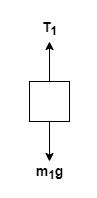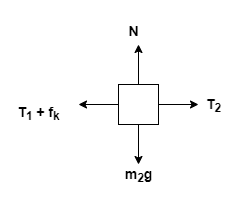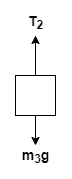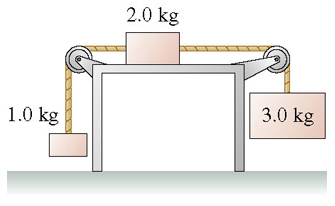# Problem: The coefficient of kinetic friction between the 2.0 kg block infigure and the table is 0.300.What is the acceleration of the 2.0 kg block?

###### FREE Expert Solution

Let's consider the following free-body diagrams.

For 1.0 kg block:For 2.0 kg block:For 3.0 kg block:Newton's second law:

$\overline{){\mathbf{\Sigma }}{\mathbf{F}}{\mathbf{=}}{\mathbf{m}}{\mathbf{a}}}$

From Newton's second law for the 1.0 kg block:

T1 - m1g = m1a

For the 2.0 kg block:

T2 - (T1 + fk) = m2a,

96% (296 ratings)###### Problem Details

The coefficient of kinetic friction between the 2.0 kg block infigure and the table is 0.300.

What is the acceleration of the 2.0 kg block?